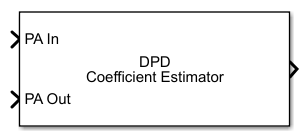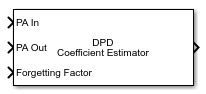# DPD Coefficient Estimator

Estimate memory-polynomial coefficients for digital predistortion

Since R2019a

•Libraries:
Communications Toolbox / RF Impairments Correction

## Description

Estimate the coefficients of a memory polynomial for digital pre-distortion (DPD) of a nonlinear power amplifier, given the baseband equivalent input and baseband equivalent output of the power amplifier. For more information, see Digital Predistortion and Optimizing Estimator Polynomial Degree and Memory Depth.

This icon shows the block with all ports enabled.## Ports

### Input

expand all

Power amplifier baseband-equivalent input, specified as a column vector.

Data Types: `double`
Complex Number Support: Yes

Power amplifier baseband-equivalent output, specified as a column vector of the same length as PA In.

Data Types: `double`
Complex Number Support: Yes

Forgetting factor used by the recursive least squares algorithm, specified as a scalar in the range (0, 1]. Decreasing the forgetting factor reduces the convergence time but causes the output estimates to be less stable.

#### Dependencies

To enable this port, set Algorithm to ```Recursive least squares``` and set Forgetting factor source to `Input port`.

Data Types: `double`

### Output

expand all

Memory-polynomial coefficients, returned as a matrix. For more information, see Digital Predistortion.

## Parameters

expand all

Desired amplitude gain in dB, specified as a scalar. This parameter value expresses the desired signal gain at the compensated amplifier output.

In addition to linearization, the DPD should make the combined gain between the DPD input and the power amplifier output as close as possible to the expected gain. Therefore, set this parameter based on the expected gain of the power amplifier that you obtain during PA characterization.

Tunable: Yes

Polynomial type used for predistortion, specified as one of these values:

• `Memory polynomial` — Computes predistortion coefficients by using a memory polynomial without cross terms

• `Cross-term memory polynomial` — Computes predistortion coefficients by using a memory polynomial with cross terms

Memory-polynomial degree, specified as a positive integer.

Memory-polynomial depth in samples, specified as a positive integer.

Adaptive algorithm used for equalization, specified as one of these values:

• `Least squares` — Estimate the memory-polynomial coefficients by using a least squares algorithm

• `Recursive least squares` — Estimate the memory-polynomial coefficients by using a recursive least squares algorithm

For algorithm reference material, see the works listed in  and .

Source of the forgetting factor, specified as one of these values:

• `Property` — Specify this value to use the Forgetting factor parameter to specify the forgetting factor.

• `Input port` — Specify this value to use the Forgetting Factor input port to specify the forgetting factor.

#### Dependencies

To enable this parameter, set Algorithm to ```Recursive least squares```.

Forgetting factor used by the recursive least squares algorithm, specified as a scalar in the range (0, 1]. Decreasing the forgetting factor reduces the convergence time but causes the output estimates to be less stable.

#### Dependencies

To enable this parameter, set Algorithm to ```Recursive least squares``` and set Forgetting factor source to `Property`.

Initial coefficient estimate for the recursive least squares algorithm, specified as a matrix of real or complex values.

• If you specify this value as an empty matrix, the initial coefficient estimate for the recursive least squares algorithm is chosen automatically to correspond to a memory polynomial that is an identity function, so that the output is equal to input.

• If you specify this value as a nonempty matrix, the number of rows must be equal to the Memory depth parameter value.

• If the Polynomial type parameter is set to `Memory polynomial`, the number of columns is the degree of the memory polynomial.

• If the Polynomial type parameter is set to `Cross-term memory polynomial`, the number of columns must equal m(n-1)+1. m is the memory depth of the polynomial, and n is the degree of the memory polynomial.

#### Dependencies

To enable this parameter, set Algorithm to ```Recursive least squares```.

Type of simulation to run, specified as `Code generation` or `Interpreted execution`.

• `Code generation` — Simulate the model by using generated C code. The first time you run a simulation, Simulink® generates C code for the block. The model reuses the C code for subsequent simulations unless the model changes. This option requires additional startup time, but the speed of the subsequent simulations is faster than with the `Interpreted execution` option.

• `Interpreted execution` — Simulate the model by using the MATLAB® interpreter. This option shortens startup time, but the speed of subsequent simulations is slower than with the ```Code generation``` option. In this mode, you can debug the source code of the block.

## Block Characteristics

 Data Types `double` | `single` Multidimensional Signals `no` Variable-Size Signals `yes`

## Algorithms

expand all

 Morgan, Dennis R., Zhengxiang Ma, Jaehyeong Kim, Michael G. Zierdt, and John Pastalan. "A Generalized Memory Polynomial Model for Digital Predistortion of Power Amplifiers." IEEE® Transactions on Signal Processing. Vol. 54, Number 10, October 2006, pp. 3852–3860.

 M. Schetzen. The Volterra and Wiener Theories of Nonlinear Systems. New York: Wiley, 1980.